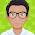# Spline Interpolation

A spline function of degree $m$ consists of polynomial functions on consequent subintervals which are connected with certain continuity conditions. In this section, three types of spline functions are considered: linear spline (degree 1), quadratic spline (degree 2) and cubic spline (degree 3, which is the most often used).

In general, a spline function can be written as:
\begin{equation} S(x) = \begin{cases} s_1(x), & x \in [x_1,x_2) \\ s_2(x), & x \in [x_2,x_3) \\ \enspace \vdots & \enspace \vdots \\ s_{n-1}(x), & x \in [x_{n-1},x_n] \end{cases} \end{equation} in which $n$ is the number of points, where $x_1 < x_2 < \cdots < x_n$, and $s_i$ is a $m$-th degree polynomial ($i = 1, 2, \cdots, n-1$) having continuous lower order derivatives.

The relevant Matlab code can be found at the end of 1-D discrete data analysis section.

#### 1 comment:

1.The equations are not showing properly. Cold you please fix this problem so that we can use the interesting content?

kind regards